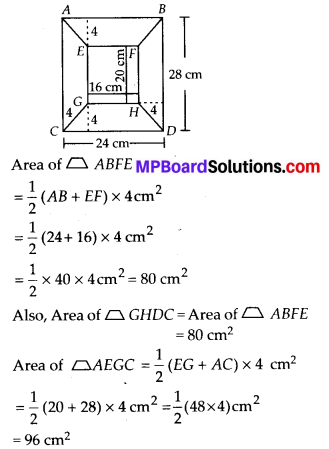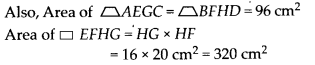## MP Board Class 8th Maths Solutions Chapter 11 Mensuration Ex 11.2

Question 1.
The shape of the top surface of a table is a trapezium. Find its area if its parallel sides are 1 m and 1.2 m and perpendicular distance between them is 0.8 m.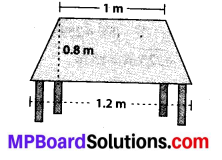Solution:
Area of trapezium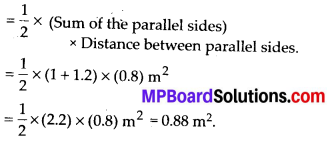Question 2.
The area of a trapezium is 34 cm2 and the length of one of the parallel sides is 10 cm and its height is 4 cm. Find the length of the other parallel side.
Solution:
Let x be the length of other parallel side.
Area of trapezium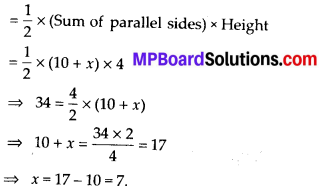∴ The length of the other parallel side is 7 cm.Question 3.
Length of the fence of a trapezium shaped field ABCD is 120 m. If BC = 48 m, CD = 17 m and AD = 40 m, find the area of this field. Side AS is perpendicular to the parallel sicles AD and BC.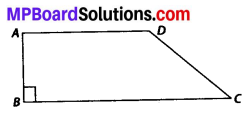Solution:
Draw a perpendicular DE from a point D which meets BC at E.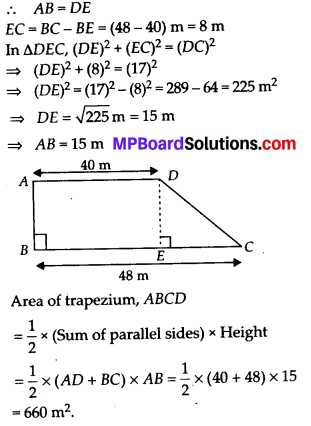Question 4.
The diagonal of a quadrilateral shaped field is 24 m and the perpendiculars dropped on it from the remaining opposite vertices are 8 m and 13 m. Find the area of the field.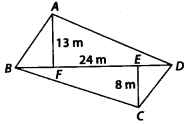Solution: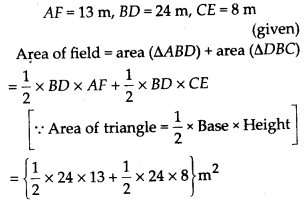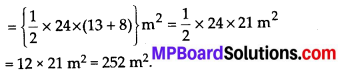Question 5.
The diagonal of a Rhombus shaped field is 7.5 cm and 12 cm. Find its area.
Solution:
We know that,
The area of a Rhombus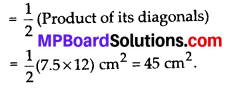Question 6.
Find the area of a rhombus whose side is 6 cm and whose altitude is 4 cm. If one of its diagonals is 8 cm long, find the length of the other diagonal.
Solution:
Since, rhombus is a parallelogram whose all sides are equal.
So, area of a rhombus = area of a parallelogram
= side × altitude = (6 × 4) cm2 = 24 cm2
Also, area of a rhombus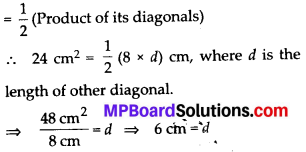∴ The length of the other diagonal be 6 cm.Question 7.
The floor of a building consists of 3000 tiles which are rhombus shaped and each of its diagonals are 45 cm and 30 cm in length. Find the total cost of polishing the floor, if the cost per m2 is ₹ 4.
Solution:
Let d1 and d2 be the diagonals of a rhombus, where d1 = 45 cm and d2 = 30 cm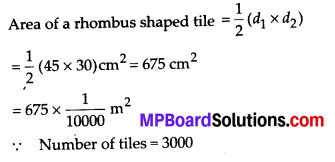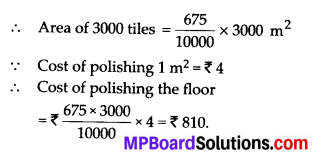Question 8.
Mohan wants to buy a trapezium shaped field. Its side along the river is parallel to and twice the side along the road. If the area of this field is 10500 m2 and the perpendicular distance between the two parallel sides is 100 m, find the length of the side along the river.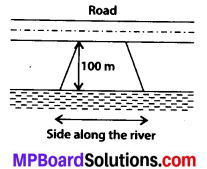Solution:
Let the opposite parallel sides of a trapezium be x and 2x.
∵ We know that,
Area of trapezium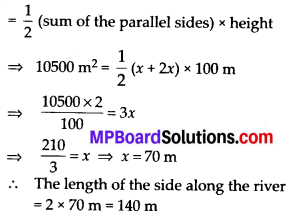Question 9.
Top surface of a raised platform is in the shape of a regular octagon as shown in the figure. Find the area of the octagonal surface.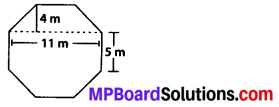Solution: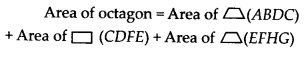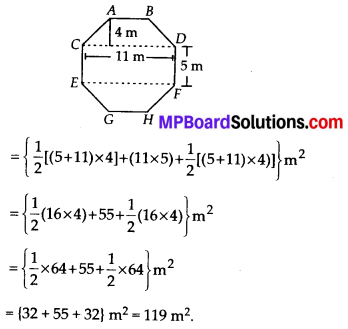Question 10.
There is a pentagonal shaped park as shown in the figure. For finding its area Jyoti and Kavita divided it in two different ways. Find the area of this park using both ways. Can you suggest some other way of finding its area ?
11-Mensuration-Ex-11.2-63.png” alt=”MP Board Class 8th Maths Solutions Chapter 11 Mensuration Ex 11.2 63″ width=”338″ height=”295″ class=”alignnone size-full wp-image-36626″ />
Solution: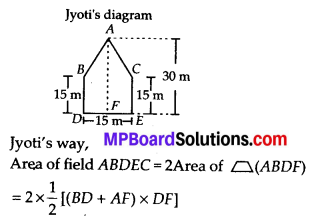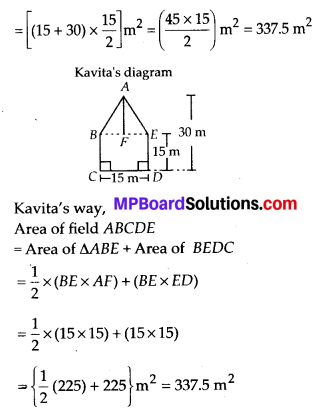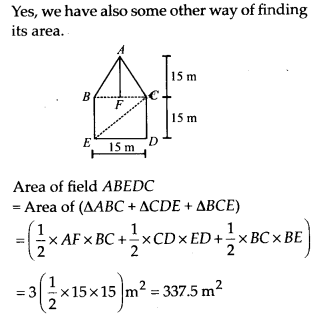Question 11.
Diagram of the adjacent picture frame has outer dimensions = 24 cm × 28 cm and inner dimensions 16 cm × 20 cm. Find the area of each section of the frame, if the width of each section is same.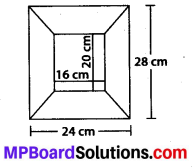Solution: